# Ex 13.1 NCERT Solutions- Surface Areas and Volumes Class 9 Notes | EduRev

## Class 9 : Ex 13.1 NCERT Solutions- Surface Areas and Volumes Class 9 Notes | EduRev

The document Ex 13.1 NCERT Solutions- Surface Areas and Volumes Class 9 Notes | EduRev is a part of the Class 9 Course Mathematics (Maths) Class 9.
All you need of Class 9 at this link: Class 9

Question 1. A plastic box 1.5 m long, 1.25 m wide and 65 cm deep is to be made. It is opened at the top. Ignoring the thickness of the plastic sheet, determine: (i) The area of the sheet required for making the box. (ii) The cost of sheet for it, if a sheet measuring 1 mcosts  20.
Solution:
(i) Here, l = 1.5 m, b = 1.25 m h = 65 cm = (65/100)
m = 0.65 m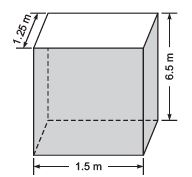∵ It is open from the top.
∵ Its surface area = [Lateral surface area] + [Base area]
= [2(l + b)h] + [l × b] = [2(1.50 + 1.25)0.65 m2] + [1.50 x 1.25 m2]
= [2 x 2.75 x 0.65 m2] + [1. 875 m2]
= 3.575 m2 + 1.875 m= 5.45 m
∵ The total surface area of the box = 5.45 m2
∴ Area of the sheet required for making the box = 5.45 m2

(ii) ∵ Rate of sheet = ₹ 20 per m2
∴ Cost of 5.45 m= ₹ 20 x 5.45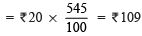⇒ Cost of the required sheet = ₹ 109

Question 2. The length, breadth and height of a room are 5m, 4m and 3m respectively. Find the cost of white washing the walls of the room and the ceiling at the rate of  7.50 per m2.
Solution:
Length of the room (l)= 5m  Breadth of the room (b) = 4 m
Height of the room (h) = 3 m
The room is like a cuboid whose four walls (lateral surface) and ceiling are to be white washed.
∴ Area for white washing = [Lateral surface area] + [Area of the ceiling]
= [2(l + b)h] + [l x b] = [2(5 + 4) x 3 m2] + [5 x 4 m2] = [54 m2] + [20 m2] = 74 m2
Cost of white washing: Cost of white washing for 1 m= ₹ 7.50
∴ Cost of white washing for 74 m2 = ₹ 7.50 x 74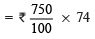=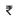555
The required cost of white washing is ₹555.

Question 3. The floor of a rectangular hall has a perimeter 250 m. If the cost of painting the four walls at the rate of 10 per m2 is 15000,
find the height of the hall.
Solution:
Note: Area of four walls = Lateral surface area.
A rectangular hall means a cubiod.
Let the length and breadth of the hall be ‘l’ and ‘b’ respectively.
∴ [Perimeter of the floor] = 2(l + b) ⇒ 2(l + b) = 250 m.
∵ Area of four walls = Lateral surface area = [2(l + b)] x h [where ‘h’ is the height of hall.]
∴ Cost of painting the four walls = ₹ 10 x 250 h = ₹ 2500 h
⇒₹2500 h = ₹ 15000
⇒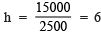Thus, the required height of the hall = 6 m

Question 4. The paint in a certain container is sufficient to paint an area equal to 9.375 m2. How many bricks of dimensions 22.5 cm x 10 cm x 7.5 cm can be painted out of this container?
Solution:
Total area that can be painted = 9.375 m2, since a brick is like a cuboid
∴ Total surface area of a brick = 2[lb + bh + hl]
= 2[(22.5 x 10) + (10 x 7.5) + (7.5 x 22.5)] cm2
= 2[(225) + (75) + (168.75)] cm
= 2[468.75] cm2
= 937.5 cm2 =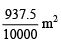Let the required number of bricks = n
∴ Total surface area of ‘n’ bricks = n x⇒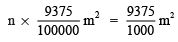⇒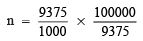⇒      n = 100
Thus, the required number of bricks = 100

Question 5. A cubical box has each edge 10 cm and another cuboidal box is 12.5 cm long, 10 cm wide and 8 cm high. (i) Which box has the greater lateral surface area and by how much? (ii) Which box has the smaller total surface area and by how much?
Solution:
For the cubical box
∵ Edge of the cubical box = 10 cm
∴ Lateral surface area = 4a2 = 4 x 102 cm2 = 4 x 100 cm2 = 400 cm2
Total surface area = 6a2 = 6 x 102 = 6 x 100 cm= 600 cm2
∵ For the cuboidal box,
l = 12.5 cm, b = 10 cm, h = 8 cm
∴ Lateral surface area = 2[(l + b)] x h = 2[12.5 + 10] x 8 cm
= 2[22.5 x 8] cm2 = 360 cm2
Total surface area = 2[lb + bh + hl]
= 2[(12.5 x 10) + (10 x 8) + (8 x 12.5)] cm2
= 2[125 + 80 + 100] cm2
= 2 cm2 = 610 cm2

Obviously, (i) ∵ 400 cm2 > 360 cm2 and 400 – 360 = 40
∴ The cubical box, has greater lateral surface area by 40 m2.

(ii) ∵ 610 cm2 > 600 cm2 and 610 – 600 = 10
∴ The cuboidal box has greater total surface area by 10 m2.

Question 6. A small indoor greenhouse (herbarium) is made entirely of glass panes (including base) held together with tape. It is 30 cm long, 25 cm wide and 25 cm high. (i) What is the area of the glass? (ii) How much of tape is needed for all the 12 edges?
Solution:
The herbarium is like a cuboid Here, l = 30 cm, b = 25 cm, h = 25 cm

(i) ∵ Area of a cuboid = 2[lb + bh + hl]
∴ Surface area of the herbarium (glass) = 2[(30 x 25) + (25 x 25) + (25 x 30)] cm2
= 2[750 + 625 + 750] cm2
= 2 cm= 4250 cm2
Thus, the required area of glass is 4250 cm2.

(ii) Total length of 12 edges = 4l + 4b + 4h = 4(l + b + h)
= 4(30 + 25 + 25) cm
= 4 x 80 cm = 320 cm
Thus, length of tape needed = 320 cm

Question 7. Shanti Sweets Stall was placing an order for making cardboard boxes for packing their sweets. Two sizes of boxes were required. The bigger of dimensions 25 cm x 20 cm x 5 cm and the smaller of dimensions 15 cm x 12 cm x 5 cm. For all the overlaps, 5% of the total surface area is required extra. If the cost of the cardboard is ₹ 4 for 1000 cm2, find the cost of cardboard required for supplying 250 boxes of each kind.
Solution:
For bigger box:
Length (l) = 25 cm,
Height (h) = 5 cm
∵ The box is like a cuboid and total surface area of a cuboid
= 2(lb + bh + hl)
∴ Area of a box = 2([25 x 20) + (20 x 5) + (5 x 25)] cm
= 2[500 + 100 + 125] cm2
= 2 cm2 = 1450 cm2
⇒ Total surface area of 250 boxes = 250 x 1450 cm2 = 362500 cm2
For smaller box:
l = 15 cm, b = 12 cm, h = 5 cm
Total surface area of a box = 2[lb + bh + hl]
= 2[(15 x 12) + (12 x 5) + (5 x 15)]cm2
= 2[180 + 60 + 75] cm2
= 2 cm2 = 630 cm2
⇒ Total surface area of 250 boxes = 250 x 630 cm2 = 157500 cm2
Now, total surface area of both kinds of boxes = 362500 cm2 + 157500 cm2
= 5,20,000 cm2
Area for overlaps = 5% of [total surface area]
= (5/100)x 520000 cm2 = 26000 cm2
∴ Total area of the cardboard required = [Total area of 250 boxes] + [5% of total surface area]
= 520000 cm2 + 26000 cm2
= 546000 cm2

Cost of cardboard:

∵   Cost of 1000 cm2 = ₹ 4

∴  Cost of 546000 cm2 = (4/546000)/ 1000

= ₹ 4*546

= ₹ 2184

Question 8. Parveen wanted to make a temporary shelter for her car, by making a box-like structure with tarpaulin that covers all the four sides and the top of the car (with the front face as a flap which can be rolled up). Assuming that the stitching margins are very small, and therefore negligible, how much tarpaulin would be required to make the shelter of height 2.5 m, with base dimensions 4 m x 3 m?
Solution:
Here, height (h) = 2.5 m
Base dimension = 4 m x 3 m
⇒ Length (l) = 4 m and
∵ The structure is like a cuboid.
∴ The surface area of the cuboid, excluding the base. = [Lateral surface area] + [Area of ceiling] = [2 (l + b)h] + [lb]
= [2 (4 + 3) x 2.5] + [4 x 3] m2
=  +  m2 = 47 m2
Thus, 47 m2 tarpaulin would be required.

SURFACE AREA OF A RIGHT CIRCULAR CYLINDER

When axis of a cylinder is perpendicular to the base radius then it is called a right circular cylinder.
If ‘r’ and ‘h’ be the radius and height of a cylinder respectively, then (i) Base area (= top area) = πr2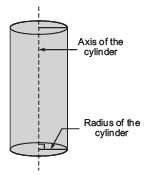(ii) Curved surface area (Lateral surface area) of a cylinder = 2πrh

(iii) Total surface area of a cylinder = 2πrh + 2πr2 = 2πr(h + r)

Note: Unless it is mentioned assume π = (22/7)

Offer running on EduRev: Apply code STAYHOME200 to get INR 200 off on our premium plan EduRev Infinity!

## Mathematics (Maths) Class 9

190 videos|233 docs|82 tests

,

,

,

,

,

,

,

,

,

,

,

,

,

,

,

,

,

,

,

,

,

;